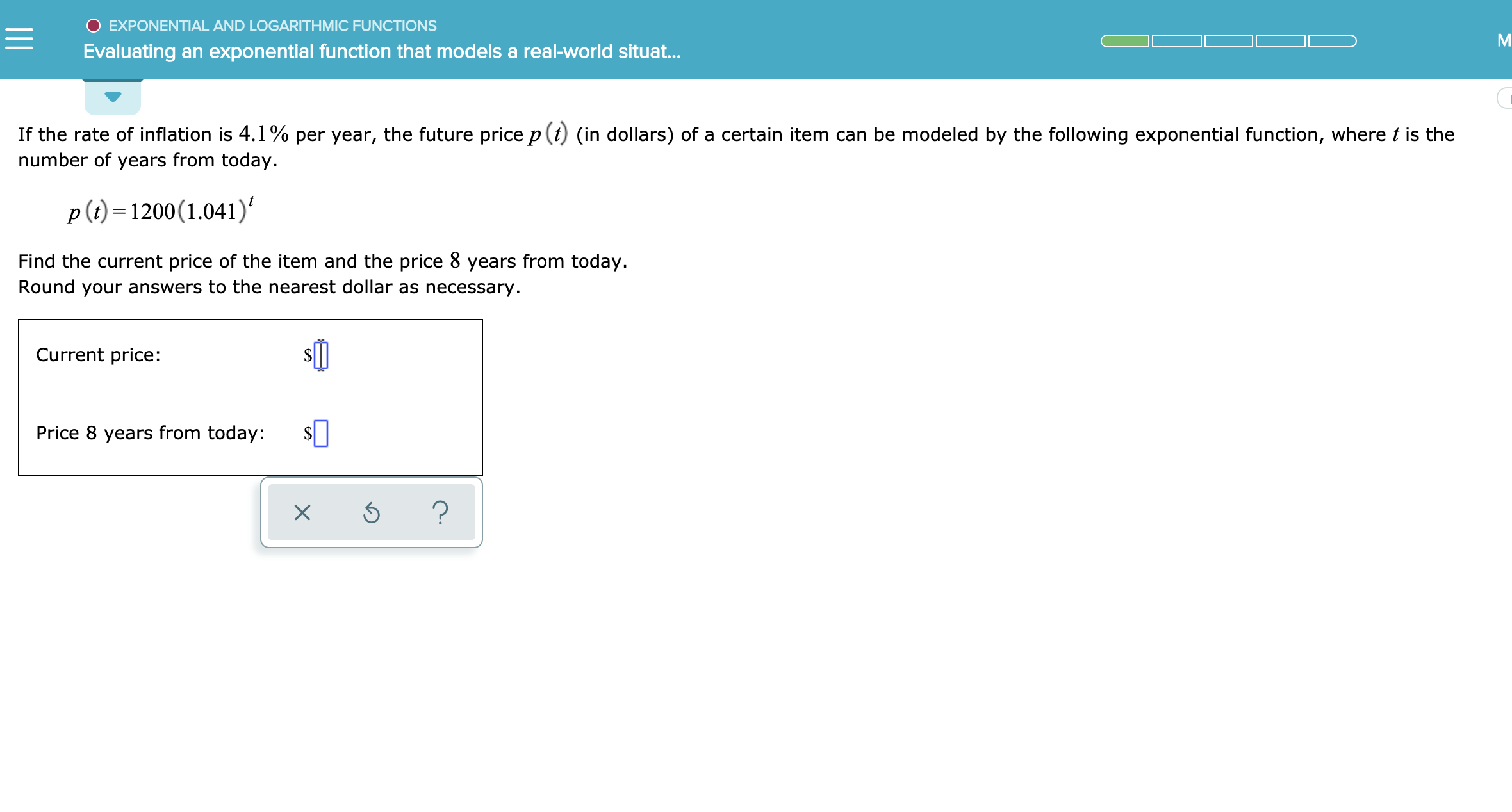# EXPONENTIAL AND LOGARITHMIC FUNCTIONSEvaluating an exponential function that models a real-world situat...If the rate of inflation is 4.1% per year, the future price p(t) (in dollars) of a certain item can be modeled by the following exponential function, where t is thenumber of years from today.p ()1200(1.041)Find the current price of the item and the price 8 years from today.Round your answers to the nearest dollar as necessary.Current price:Price 8 years from today:?X

Question
18 views

See attachedhelp_outlineImage TranscriptioncloseEXPONENTIAL AND LOGARITHMIC FUNCTIONS Evaluating an exponential function that models a real-world situat... If the rate of inflation is 4.1% per year, the future price p(t) (in dollars) of a certain item can be modeled by the following exponential function, where t is the number of years from today. p ()1200(1.041) Find the current price of the item and the price 8 years from today. Round your answers to the nearest dollar as necessary. Current price: Price 8 years from today: ? X fullscreen
check_circle

Step 1

The given exponential model of future price, p (in dollars) as a function of time t is given below.

Step 2

The current price of the item is given by plugging the value of t = 0 in the above function.

Step 3

The price of the item, 8 years from today is given by plugging...

### Want to see the full answer?

See Solution

#### Want to see this answer and more?

Solutions are written by subject experts who are available 24/7. Questions are typically answered within 1 hour.*

See Solution
*Response times may vary by subject and question.
Tagged in

### Other